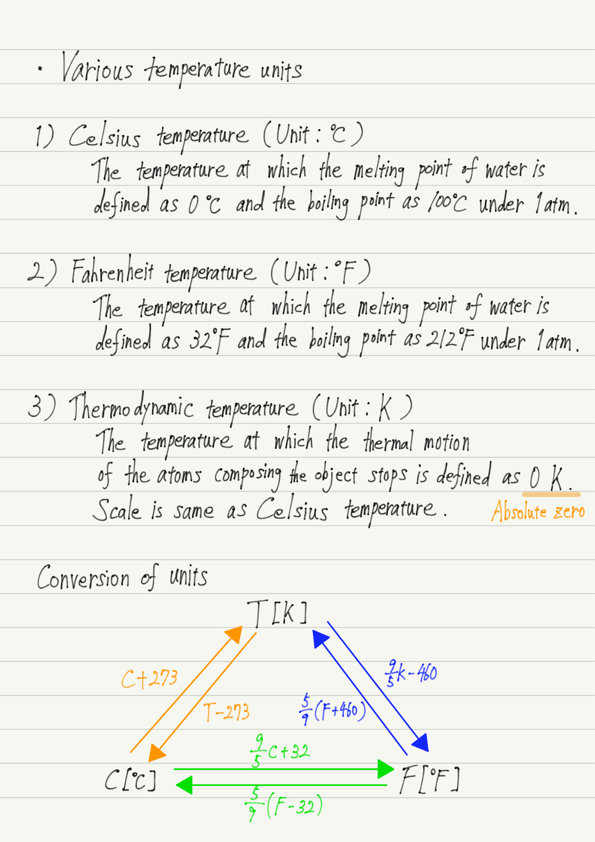# Various Temperature Units

In this lecture we will look more closely at temperature.

Temperature is a numerical expression of the intensity of thermal motion, and there are many ways to express it.
We would like to present some commonly used temperatures.

## 1. Celsius temperature (℃)

First is the Celsius temperature.
This is the temperature determined by Celsius based on the phase transition of water and is expressed in °C.
Specifically, the temperature at which ice melts under 1 atmospheric pressure is defined as 0°C, and the temperature at which water boils is defined as 100°C.

## 2. Fahrenheit temperature(℉)

Next is the Farenheit temperature, which is measured in ℉.
It is often used mainly in the United States.
This is the temperature at which ice melts at 32℉ and the temperature at which it boils at 212℉.
There are many theories as to why the reference number is in the middle of the range, but one advantage of using Farenheit temperatures is that they require almost no minus when expressing atmospheric temperature.

## 3. Thermodynamic temperature(K)

Temperature is determined by the intensity of thermal motion of atoms and molecules.
As an object gets cooler and cooler, the thermal motion becomes slower and slower.
Still, if you continue to cool it down, eventually the thermal motion will stop (actually, it will not stop completely, but don’t worry about that for now).

Since temperature is determined by the intensity of thermal motion, once thermal motion stops, the temperature cannot fall below that level.

Therefore, the temperature at which the thermal motion of the atoms composing the object stops is set to 0, and the temperature determined by dividing it by the same scale as the Celsius temperature is called the thermodynamic temperature.
The unit for thermodynamic temperature is K (Kelvin).

0K is -273°C in Celsius temperature and -460°F in Fahrenheit temperature, and is also called absolute zero because there is no temperature below this temperature.

Thermodynamic temperature is very commonly used in physics because it is based on the essence of temperature: thermal motion.
However, while useful in physics, the numbers are too large and confusing to use in everyday life.
Therefore, we are forced to distinguish between thermodynamic temperatures and familiar temperatures.

Refer to the summary for calculation methods.

## Summary of this lecture## Next Time

Learn about the relationship between heat and temperature.

https://www.yukimura-physics.com/entry/therm-f03

error: Content is protected !!